• 2021-04-15 20:11:23

本文主要向大家介绍了C语言编程入门的求一元二次方程的根，通过具体的代码向大家展示，希望对大家学习C语言编程入门有所帮助。

分析：

1.  当a = 0： 不是一个一元二次方程；

2.  当a！=0： (1)当b^2-4ac=0,方程有两个相等的实根，x1=x2=-b/2a；

(2)当b^2-4ac>0,方程有两个不相等的实根,x1=(-b+√(b^2-4ac))/(2a), x2=(-b-√(b^2-4ac))/(2a)；

(3)当b^2- 4ac<0，方程有一组共轭复根；

3.方程的根在很多情况下是小数，因此在定义变量时不能用整型，可以用浮点型或者double型；

4.对于变量与零值进行比较时，不能讲浮点变量用“==”或“!=”与任何数字进行比较，可以使用#define 定义一个精度，在使用时只要在给定的范围内，将其近似为0；

5.使用函数sqrt必须引用头文件#include。

程序代码如下：[cpp] view plain copy

1. #include

2. #include

3. #define EXP 0.00000001

4. int main()

5. {

6.     double a = 0.0;

7.     double b = 0.0;

8.     double c = 0.0;

9.     scanf_s("%lf%lf%lf",&a,&b,&c);

10.     if ((a>=-EXP)&&(a<=EXP))

11.     {

12.         printf("不是一元二次方程\n");

13.     }

14.     else

15.     {

16.         double disc = b*b-4*a*c;

17.

18.         if(( disc >= -EXP)&&( disc <= EXP))

19.         {

20.             printf("x = %lf\n",-b/(2*a));

21.         }

22.         else if ( disc > 0.0)

23.         {

24.             printf("x1 = x2 %lf, x2 = %lf\n",

25.                 (-b+sqrt(disc))/(2*a),

26.                 (-b-sqrt(disc))/(2*a));

27.         }

28.         else

29.         {

30.             printf("有两个共轭的复根\n");

31.         }

32.     }

33.

34.     return 0;

35. }

本文由职坐标整理并发布，希望对同学们有所帮助。了解更多详情请关注职坐标编程语言C/C+频道！

更多相关内容
• 主要介绍了Python编程实现数学运算求一元二次方程的实算法,涉及Python数学运算求解方程的相关实现技巧,需要的朋友可以参考下
• 最简单的一元二次方程求根问题的C源码，小白易懂，忽略了简洁好看，突出易懂，已在Devc++和VC++成功运行。
• 一元二次方程 ax2+bx+c=0ax^2+bx+c=0ax2+bx+c=0，a、b、c 的值由用户在三行中输入，根据用户输入的数值求解方程的实数解： 如果 a 值为0，根据 b 值判断方程是否有解并输出（若有解，输出保留两位小数），如果 a 与...

# 题目描述

一元二次方程 a x 2 + b x + c = 0 ax^2+bx+c=0 ，a、b、c 的值由用户在三行中输入，根据用户输入的数值求解方程的实数解：

• 如果 a 值为0，根据 b 值判断方程是否有解并输出（若有解，输出保留两位小数），如果 a 与 b 同时为 0，则输出 Date error!
• 如果方程无实数解，输出“该方程无实数解”；
• 如果方程有两个相同的实数解，输出一个解，结果保留 2 位小数；
• 如果方程有两个不同的实数解，在一行内按从小到大顺序输出方程的两个解，用空格分隔，结果保留 2 位小数。

# 输入格式

输入三行数据，每行输入一个实数。

方程的解。

# 测试样例

## 样例1

### 输入

8
5
2


### 输出

该方程无实数解


## 样例2

### 输入

0
0
9


### 输出

Data error!


## 样例3

### 输入

3
6
2


### 输出

-0.42 -1.58


# 参考代码

class Solution:
def __init__(self):
self.a = eval(input())
self.b = eval(input())
self.c = eval(input())

def __main__(self) -> str:
d = self.b**2-4*self.a*self.c
if self.a == 0 and self.b == 0:
return 'Data error!'
elif self.a == 0 and self.b != 0:
return f'{-self.c/self.b:.2f}'
else:
if d > 0:
x1 = (-self.b+d**0.5)/(2*self.a)
x2 = (-self.b-d**0.5)/(2*self.a)
x1, x2 = (x2, x1) if x1 < x2 else (x1, x2)
return f'{x1:.2f} {x2:.2f}'
elif d == 0:
return f'{-self.b/(2*self.a):.2f}'
else:
return '该方程无实数解'

if __name__ == '__main__':
print(Solution().__main__())

展开全文python
• vb小程序，一元次方程求根计算。用Visual Basic开发的
• Labview编写的进行一元二次方程的计算，可设置不同的系数进行计算。labview
• 用python求一元二次方程式的

一、算法的构思
一元二次方程的一般式：ax^2+bx+c = 0
我们知道，一元二次方程有解（根）的充要条件是：b^2-4ac>=0。如果不满足此关系式，那么方程无解。接着当方程有解的时候又出现了两种情况：1.有两个重根（大小相等的根）或者两个大小不等的根，为了是程序更加完善还要考虑到a =0的情况，即此时不能看做一元二次方程而只能将其看作一元一次方程，本程序运用求根公式来实现功能，有兴趣的伙计可以试试下面给出的韦达定理实现功能。
求根公式：二、全部代码

'''
1.本程序用来解决一元二次方程在实数域上求根的问题
2.可按需求最大/最小值及对称轴，
3.可以绘制函数图像
'''
'''
.duichenzhou属性用来求解对称轴x轴坐标
.max_min属性用来求函数最大最小值
.x1/x2属性用来求解方程的两个根
######
使用方法
1.首先调用.calculate(a,b,c)用来计算
2.调用完成后即可调用相关属性
3.可选择.visual_image()方法进行可视化
'''
import matplotlib.pyplot as plt
import numpy as np

class L2_fun(object):
def __init__(self):
self.flag = True
self.duichenzhou = 0.0
self.max_min = 0.0
self.x1 = 0.0
self.x2 = 0.0
def calculate(self,a,b,c):
if a==0:
self.x1 = (-c)/b
self.x2 = self.x1
elif (b**2-4*a*c)>=0:
self.duichenzhou = (-b)/(2*a)
self.x1 = (-b+np.sqrt(b**2-4*a*c))/(2*a)
self.x2 = (-b-np.sqrt(b**2-4*a*c))/(2*a)
if a>0:
self.max_min = (4*a*c-b*b)/(4*a)
print('提示：此函数开口为上，极值获取的是最小值！\n')
if a<0:
self.max_min = (4*a*c - b*b) /(4*a)
print('提示：此函数开口为下，获取的是最大值！\n')
else:
self.flag = False
self.duichenzhou = (-b)/(2 * a)
self.max_min = (4*a*c-b*b)/(4*a)
print('此方程在实数域上无解！！！\n')
self.x1=self.x2 ='无解！'
def visual_image(self,a,b,c):
if self.flag==False:
print('此方程无解，但可可视化图像！\n')
v = int(self.duichenzhou)
x = np.linspace(v-15,v+15,2800)
y = a*(x**2)+b*x+c
plt.figure(figsize=(12,8),dpi=100)
plt.title('y = {}*(x**2)+{}*(x)+{}'.format(a,b,c),color ='r',fontsize=22)
plt.xlabel('x',loc= 'right',fontsize = 18)
plt.ylabel('y',loc='top',fontsize = 18)
plt.plot(x,y,color='c',label = 'y')
plt.grid(alpha=0.3,linestyle ='--')
plt.legend(loc = 'best')
plt.show()
if __name__ == '__main__':
a,b,c = map(float,input('请以空格分隔输入3个数字，免费进行运算！\n').split())#split默认以空格进行分隔
S = L2_fun()
S.calculate(a,b,c)
print(S.duichenzhou,S.x2)
S.visual_image(a,b,c)


三、需要用到的库(必须安装)
请安装numpy、和matplotlib库
将下面两句分别复制到cmd命令中，回车运行：
pip install numpy -i https://pypi.tuna.tsinghua.edu.cn/simple
pip install matplotlib -i https://pypi.tuna.tsinghua.edu.cn/simple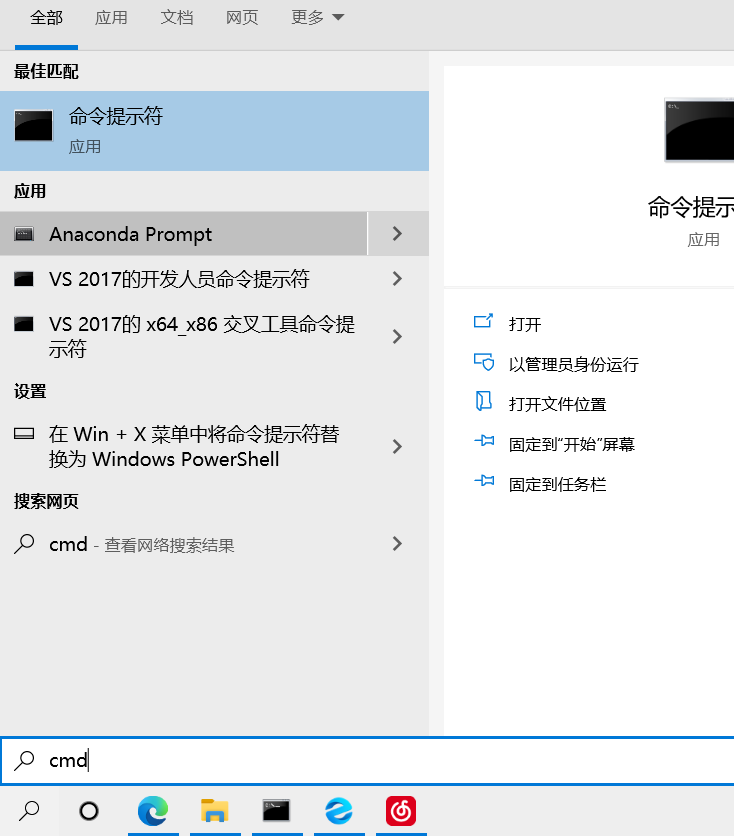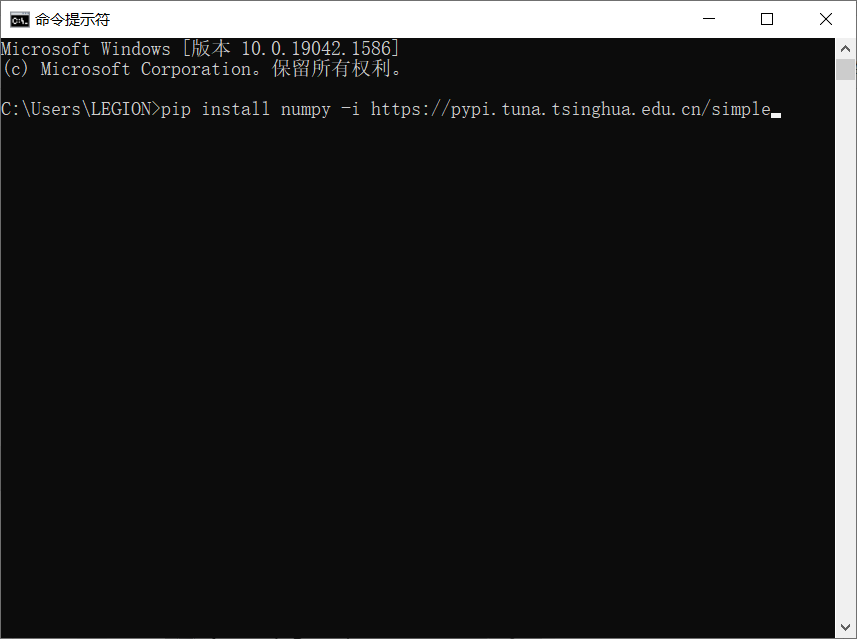四、效果演示
欢迎大家点赞，批评指正，谢谢！！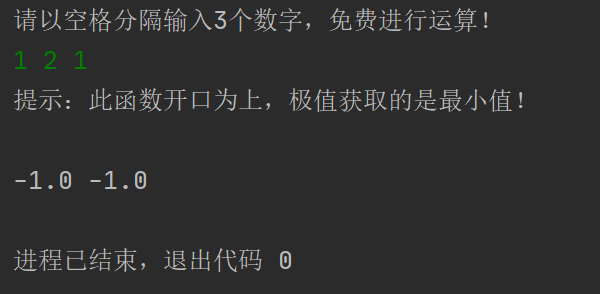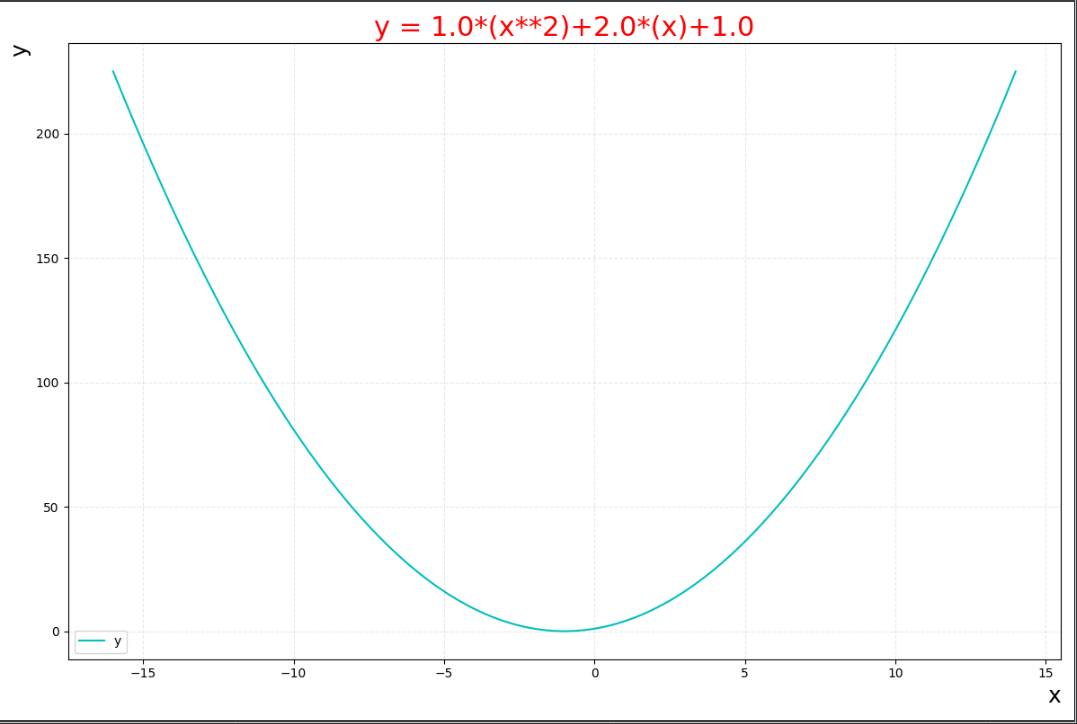展开全文python 算法 学习
• //// main.c// 一元二次方程求解//// Created by 丁小未 on 13-7-9.// Copyright (c) 2013年 dingxiaowei. All rights reserved.//#include #include float O(float a,float b,float c){float res = b*b-4*a*c;if...

//

// main.c

// 一元二次方程求解

//

// Created by 丁小未 on 13-7-9.

//

#include

#include

float O(float a,float b,float c)

{

float res = b*b-4*a*c;

if(res<0)

{

return -1;

}

else

{

return res;

}

}

int main(int argc, const char * argv[])

{

float a,b,c;

char flag='y';

printf("*******欢迎使用一元二次方程求根系统***************\n");

do

{

do {

printf("\n`请输入一元二次方程的a,b,c参数(例如1,4,4)：");

scanf("%f,%f,%f",&a,&b,&c);

} while (0==a);

float res;

float x,y;

res=O(a, b, c);

if (res<0)

{

printf("方程无解!");

}

if(b*b==4*a*c)

{

x=(-b)/2*a;

printf("方程的解：X=Y=%.2f",x);

}

if(res>0)

{

x=(-b+sqrtf(res))/2*a;

y=(-b-sqrtf(res))/2*a;

printf("方程的解:\nX=%.2f\nY=%.2f",x,y);

}

printf("\n您想继续吗？(y/n)");

flag = getchar(); //要输入两次是清除之前获取到的留在缓存中的'\n'

flag = getchar();

}while ('y'==flag);

return 0;

}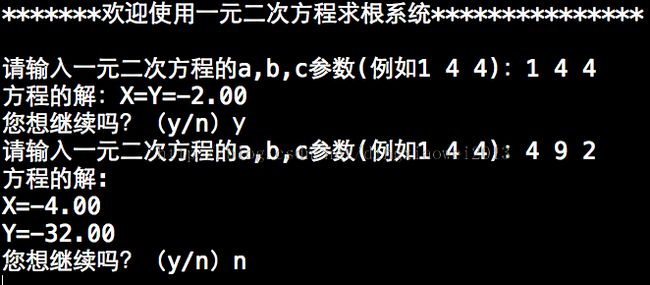展开全文• 本文实例讲述了Python实现求解一元二次方程的方法。分享给大家供大家参考，具体如下： 1. 引入math包 2. 定义返回的对象 3. 判断b*b-4ac的大小 具体计算代码如下： # -*- coding:utf-8 -*- #! python2 import math ...
• 【提示】要考虑各种情形:一是 a 为0的情形,方程为-c/......(理论考试重点内容实践) 一元二次方程白盒测试要求 1) 自备 C 语言程序(可从 C 语言程序设计的教科书上摘录若干典型程序,其他 C++、C#、Java 程序均可);...
•c语言
• = 0: delta = b**2-4*a*c if delta  print("无根") elif delta == 0: s = -b/(2*a) print("唯一的x=",s) else: root = math.sqrt(delta) x1 = (-b+root)/(2*a) x2 = (-b-root)/(2*a) print("x1=",x1,"\t","x2=",...python 开发语言 后端
• 求根公式解一元二次方程 import math def quadratic(a, b, c): if a == 0: if b == 0: if c == 0: print('方程有任意解') else: print('方程无解') else: x = -c / b print('方程有解：x=%.2f'python
• ## Python一元二次方程求根

万次阅读 多人点赞 2018-09-18 17:36:34
Python一元二次方程求根 1、任务简介 在之前的博客中我分享了使用Java进行一元二次方程求根的方法，在学习了Python之后我也想使用Python编写一个类似的程序，故在编写成功后将该任务分享出来。 2、任务代码 ...Python
• Here you will get C program to find roots of quadratic equationax2+bx+c=0 在这里，您将获得C程序来查找二次方程ax 2 + bx + c = 0的 #include<stdio.h> #include<math.h> int main() { float ...python 算法 linux c++
• 对于简单的一元次方程的迭代#include #include #include #define MAXTIMES 5typedef int times;typedef double coefficient;typedef struct _properties{coefficient x; //系数times n;//次数}...
•python 开发语言
• 从键盘上输入a,b,c的值，编程计算并输出一元二次方程 一.无要求 代码如下: #include<stdio.h> #include<math.h> int main() { double a, b, c, x1, x2; printf("请输入三个系数a,b,c\n"); ...c语言 学习
• java求一元二次方程关注:168答案:2mip版解决时间 2021-02-03 01:41提问者青春统帅2021-02-02 10:42out;+k);if(p&gt.in);方程只有一个跟"System.nextDouble()import java.println(&quot.sqrt(p))/.out....
• /*包含stdio.h头文件，以便于使用输入函数scanf和输出函数printf*/ .../*定义一元二次方程的系数 ax^2 + bx +c =0*/ floata,b,c; /*定义变量deta = b*b - 4ac*/ floatdelta; /*定义两个*/ fl...c语言
• // 一元二次方程 for (int i = 0; i < 10; i++) { Console.Title = "一元二次方程程序"; double a, b, c, d, x1, x2; Console.WriteLine("求解一元二次方程ax*x+bx+c=0"); Console.WriteLine("请输入a：")...c# linq 开发语言
• //求解一元二次方程ax^2+bx+c=0的实和复根，Test类是主类class OnceBasicQuadraticEquation //求解一元二次方程ax^2+bx+c的实和复根的类，其中getRoot()方法求根和显示{private double a; //方程的二次项系数...
• 利用C++求一元二次方程，不能单纯地依靠数学原理，否则会得出错误的结果！ 先看一道例题： 1058：求一元二次方程 时间限制: 1000 ms 内存限制: 65536 KB 提交数: 102128 通过数: 18399 【题目描述】 利用...c++ 开发语言 后端 c语言
• 从键盘任意输入a，b，c的值，编程计算并输出一元二次方程ax2+bx+c=0的。根据一元二次方程求根公式，令 , 当b2−4ac=0时，输出两个相等的实x1=x2=p；当b2−4ac>0时，输出两个不相等的实：x1=p+q，x2=p−q...c语言
• 　编写一个求解一元二次方程的实数的程序，方程的系数由用户在运行xh 输入格式 　输入一行三个整数分别为一元二次方程的三个系数，数据之间以空格隔开 输出格式 　输出一行为方程的实数（若两不同 较大的在前 ...
• 求一元二次方程 程序流程图： 代码： #include<stdio.h> #include<math.h> void main() { float a,b,c,s,x1,x2; printf("please in put a b c:\n"); scanf("%f,%f,%f",&a,&b,&c); if...c语言...